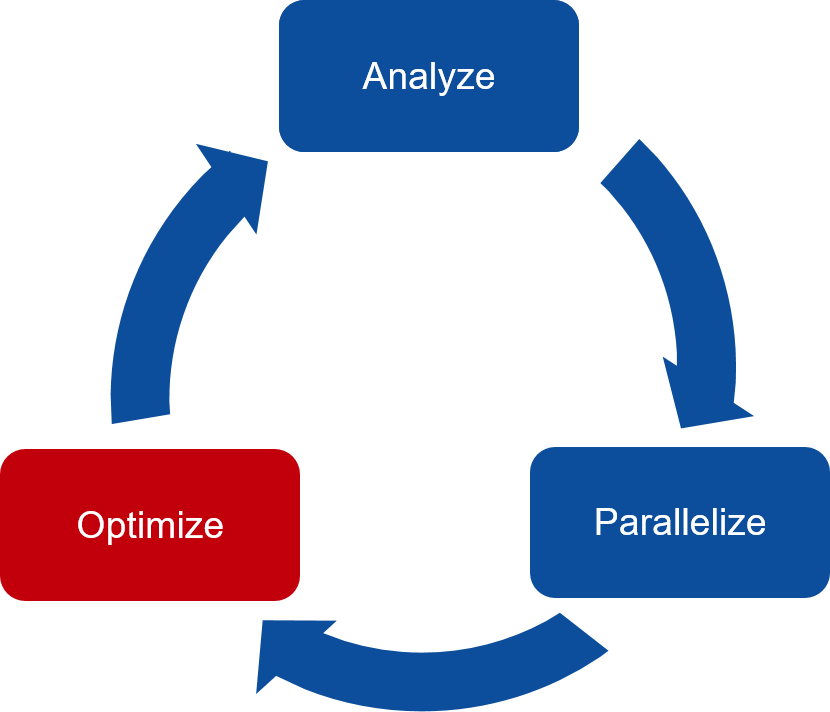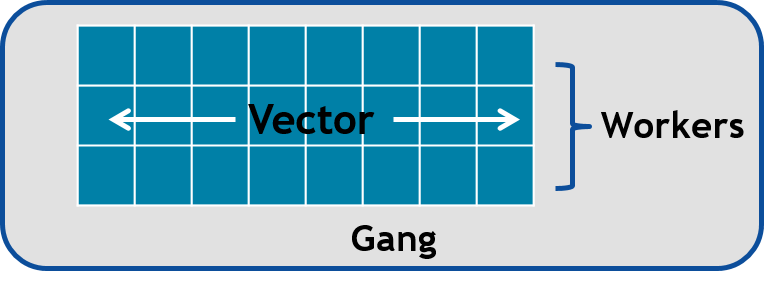# Introduction to OpenACC (cont.)¶

• The three key steps in porting to high performance accelerated code:

1. Analyze/Identify parallelism

2. Express data movement and parallelism

3. Optimize data movement and loop performance

4. Go back to 1!• Analyze your code to determine most likely places needing parallelization or optimization.

• Parallelize your code by starting with the most time consuming parts and check for correctness.

• Optimize your code to improve observed speed-up from parallelization.

## Otimizing data movement¶

• Minimize the data transfer between host and device

• Constructs and clauses for

• defining the variables on the device

• transferring data to/from the device

• All variables used inside the `parallel` or `kernels` region will be treated as implicit variables if they are not present in any data clauses, i.e. copying to and from to the device is automatically performed

```// #pragma acc data copy(vecA,vecB,vecC)
#pragma acc kernels
for (i = 0; i < NX; i++) {
vecC[i] = vecA[i] * vecB[i];
}
```
```\$ pgcc -g -O3 -acc -Minfo=accel -ta=nvidia sum.c -o sum
23, Generating implicit copyout(vecC[:]) [if not already present]
Generating implicit copyin(vecB[:],vecA[:]) [if not already present]

\$ export PGI_ACC_TIME=1
\$ ./sum
time(us): 247
23: data region reached 2 times
23: data copyin transfers: 2
device time(us): total=174 max=99 min=75 avg=87
25: data copyout transfers: 1
device time(us): total=73 max=73 min=73 avg=73

#### previous with explicit data copy
Accelerator Kernel Timing data
main  NVIDIA  devicenum=0
time(us): 451
21: data region reached 2 times
21: data copyin transfers: 3
device time(us): total=245 max=100 min=71 avg=81
29: data copyout transfers: 3
device time(us): total=206 max=72 min=67 avg=68
```
• Typically data on the device has the same lifetime as the OpenACC construct (`parallel`, `kernels`, `data`) it is declared in

• It is possible to declare and refer to data residing statically on the device until deallocation takes place

## Data constructs: data clauses¶

• `copy(var-list)` on entry: if data is present on the device on entry, behave as with the present clause, otherwise allocate memory on the device and copy data from the host to the device. on exit: copy data from the device to the host and deallocate memory on the device if it was allocated on entry

• `copyin(var-list)` on entry: same as copy on entry, on exit: deallocate memory on the device if it was allocated on entry

• `copyout(var-list)` on entry: if data is present on the device on entry, behave as with the present clause, otherwise allocate memory on the device on exit: same as copy on exit

• `present(var-list)` on entry/exit: assume that memory is allocated and that data is present on the device

• `create(var-list)` on entry: allocate memory on the device, unless it was already present, on exit: deallocate memory on the device if it was allocated on entry

• `reduction(operator:var-list)` the operator can be `+,-,*,max,min`, Performs reduction on the (scalar) variables in list

### Data specification¶

• Data clauses specify functionality for different variables

• Overlapping data specifications are not allowed

• For array data, array ranges can be specified

• C/C++: `arr[start_index:length]`, for instance `vec[0:n]`

• Fortran: `arr(start_index:end_index)`, for instance `vec(1:n)`

• Note: array data must be contiguous in memory (vectors, multidimensional arrays etc.)

### Default data environment in compute constructs¶

• All variables used inside the `parallel` or `kernels` region will be treated as implicit variables if they are not present in any data clauses, i.e. copying to and from the device is automatically performed

• Implicit array variables are treated as having the `copy` clause in both cases

• Implicit scalar variables are treated as having the

• `copy` clause in `kernels`

• `firstprivate` clause in `parallel`

### Unstructured data regions¶

• Unstructured data regions enable one to handle cases where allocation and freeing is done in a different scope

• Useful for e.g. C++ classes, Fortran modules

• `enter data` defines the start of an unstructured data region

• C/C++: `#pragma acc enter data [clauses]`

• Fortran: `!\$acc enter data [clauses]`

• [clauses] can be `create(var-list)` to allocate memory on the device or `copyin(var-list)` to allocate memory on the device and copy data from the host to the device

• `exit data` defines the end of an unstructured data region

• C/C++: `#pragma acc exit data [clauses]`

• Fortran: `!\$acc exit data [clauses]`

• [clauses] can be `delete(var-list)` to deallocate memory on the device or `copyout(var-list)` to Deallocate memory on the device and copy data from the device to the host

### Data directive: update¶

• Define variables to be updated within a data region between host and device memory

• C/C++: `#pragma acc update [clauses]`

• Fortran: `!\$acc update [clauses]`

• Data transfer direction controlled by `host(var-list)` or `device(var-list)` clauses

• `self` (`host`) clause updates variables from device to host

• `device` clause updates variables from host to device

• `update` is a single line executable directive

• Useful for producing snapshots of the device variables on the host or for updating variables on the device

• Pass variables to host for visualization

• Communication with other devices on other computing nodes

• Often used in conjunction with

• Asynchronous execution of OpenACC constructs

• Unstructured data regions

### Data directive: declare¶

• Makes a variable resident in accelerator memory

• Added at the declaration of a variable

• Data life-time on device is the implicit life-time of the variable

• C/C++: `#pragma acc declare [clauses]`

• Fortran: `!\$acc declare [clauses]`

• Supports usual data clauses, and additionally

• `device_resident`

• `link`

## Data construct: example¶

```const int N=100;
#pragma acc data copy(a[0:N])
{
#pragma acc parallel loop present(a)
for (int i=0; i<N; i++)
a[i] = a[i] + 1;
}

...
#pragma acc data copyout(a[0:N]), copyin(b[0:N])
{
#pragma acc parallel loop present(a,b)
for (int i=0; i<N; i++)
a[i] = b[i] + 1;
}

...
#pragma acc data copyout(a[0:N]), create(b[0:N])
{
#pragma acc parallel loop
for (int i=0; i<N; i++)
b[i] = i * 2.0;

#pragma acc parallel loop present(a,b)
for (int i=0; i<N; i++)
a[i] = b[i] + 1;
}
```

## Data construct: Heat equation¶

```for (int n = 0; n < numSteps; n++)
{
/// Going through the entire area
#pragma acc parallel loop collapse(2) copyin(Un[0:nx][0:ny]) copyout(Unp1[0:nx][0:ny])
for (int i = 1; i < nx-1; i++)
{
for (int j = 1; j < ny-1; j++)
{
float uij = Un[i][j];
// Explicit scheme
Unp1[i][j] = uij + a * dt * ( (Un[i-1][j] - 2.0*uij + Un[i+1][j])/dx2
+ (Un[i][j-1] - 2.0*uij + Un[i][j+1])/dy2 );
}
}
```
```  time(us): 16,877
93: data region reached 1000 times
42: kernel launched 1000 times
grid:   block: 
elapsed time(us): total=12,437 max=50 min=11 avg=12
93: data copyin transfers: 500
device time(us): total=8,572 max=45 min=16 avg=17
107: data copyout transfers: 500
device time(us): total=8,305 max=23 min=16 avg=16
```
``` #pragma acc data copyin(Un[0:nx][0:ny]) create(Unp1[0:nx][0:ny])
{
for (int n = 0; n < numSteps; n++)
{
// Going through the entire area
#pragma acc parallel loop collapse(2)
for (int i = 1; i < nx-1; i++)
for (int j = 1; j < ny-1; j++)
Unp1[i][j] = ...

if (n % outputEvery == 0)  {
#pragma acc update host(Un[0:nx][0:ny])
save_png(Un, nx, ny, filename, 'c');
}
#pragma acc parallel loop collapse(2)
for (int i = 1; i < nx; i++)
{
for (int j = 1; j < ny; j++)
Un[i][j] = Unp1[i][j];
}
}
}
```
```        time(us): 151
93: data region reached 2 times
42: kernel launched 2 times
grid:   block: 
elapsed time(us): total=72 max=49 min=23 avg=36
93: data copyin transfers: 1
device time(us): total=45 max=45 min=45 avg=45
115: update directive reached 5 times
115: data copyout transfers: 5
device time(us): total=106 max=22 min=20 avg=21
```

## Optimize Loop performance¶

• The compiler is usually pretty good at choosing how to break up loop iterations to run well on parallel accelerators.

• Sometimes we can obtain a little more performance by guiding the compiler to make specific choices.

### Vectors, Workers, and Gangs¶

• OpenACC has three levels of parallelism

• Vector threads work in SIMT (SIMD) fashion

• Workers compute a vector

• Gangs have one or more workers that share resources, such as streaming multiprocessor - Multiple gangs work independently

• By default, when programming for a GPU, gang and vector parallelism is automatically applied.This image represents a single gang. When parallelizing our for loops, the loop iterations will be broken up evenly among a number of gangs. Each gang will contain a number of threads. These threads are organized into blocks. A worker is a row of threads. In the above graphic, there are 3 workers, which means that there are 3 rows of threads. The vector refers to how long each row is. So in the above graphic, the vector is 8, because each row is 8 threads long.

```#pragma acc parallel num_gangs( 2 ) num_workers( 4 ) vector_length( 32 )
{
#pragma acc loop gang worker
for(int i = 0; i < N; i++)
{
#pragma acc loop vector
for(int j = 0; j < M; j++)
{
< loop code >
}
}
}
```
• Avoid Wasting Threads, when parallelizing small arrays, you have to be careful that the number of threads within your vector is not larger than the number of loop iterations.

```#pragma acc kernels loop gang
for(int i = 0; i < 1000000000; i++)
{
#pragma acc loop vector(256)
for(int j = 0; j < 32; j++)
{
< loop code >
}
}

...

#pragma acc kernels loop gang worker(8)
for(int i = 0; i < 1000000000; i++)
{
#pragma acc loop vector(32)
for(int j = 0; j < 32; j++)
{
< loop code >
}
}
```
• The Rule of 32 (Warps): The general rule of thumb for programming for NVIDIA GPUs is to always ensure that your vector length is a multiple of 32 (which means 32, 64, 96, 128, … 512, … 1024… etc.). This is because NVIDIA GPUs are optimized to use warps. Warps are groups of 32 threads that are executing the same computer instruction.

### Collapse Clause¶

• The collapse clause allows us to transform a multi-dimensional loop nest into a single-dimensional loop. This process is helpful for increasing the overall length (which usually increases parallelism) of our loops, and will often help with memory locality.

```#pragma acc parallel loop
for (int i = 1; i < nx-1; i++)
{
#pragma acc loop
for (int j = 1; j < ny-1; j++)
{
Unp1[i][j] = ...

\$ pgcc ... heat_equation_openacc_1.c
97, #pragma acc loop gang /* blockIdx.x */
100, #pragma acc loop vector(128) /* threadIdx.x */

\$ PGI_ACC_TIME=1 ./heat
93: compute region reached 500 times
93: kernel launched 500 times
grid:   block: 
elapsed time(us): total=23,929 max=74 min=40 avg=47
```
```#pragma acc parallel loop collapse(2)
for (int i = 1; i < nx-1; i++)
{
for (int j = 1; j < ny-1; j++)
{
Unp1[i][j] = ...

\$ pgcc ... heat_equation_openacc_2.c
97, #pragma acc loop gang, vector(128) collapse(2) /* blockIdx.x threadIdx.x */
99,   /* blockIdx.x threadIdx.x collapsed */

\$ PGI_ACC_TIME=1 ./heat
93: compute region reached 500 times
93: kernel launched 500 times
grid:   block: 
elapsed time(us): total=22,642 max=63 min=38 avg=45
```

Explore the code in the subfolders `jacobi/`, `pi_monte_carlo/` and `HeatEquation/` and try to identify possible parallel regions for GPU offloading. Pick one of the codes and try to implement directives. Compile either by `./compile.sh` (on Tetralith) or `make` on Colab, and run by either `sbatch job.sh` (on Tetralith) or `./name-of-executable` (on Colab).

Keypoints

• Optimizing data movement:

• Data directive

• Enter data & exit data

• Unstructured data region

• Update directive

• Declare directive

• Optimizing loop performance:

• loop gang worker vector

• collapse